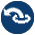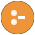#lists.pl -- List Manipulation

This library provides commonly accepted basic predicates for list manipulation in the Prolog community. Some additional list manipulations are built-in. See e.g., memberchk/2, length/2.

The implementation of this library is copied from many places. These include: "The Craft of Prolog", the DEC-10 Prolog library (LISTRO.PL) and the YAP lists library. Some predicates are reimplemented based on their specification by Quintus and SICStus.

Compatibility
- Virtually every Prolog system has library(lists), but the set of provided predicates is diverse. There is a fair agreement on the semantics of most of these predicates, although error handling may vary.
member(?Elem, ?List)
True if Elem is a member of List. The SWI-Prolog definition differs from the classical one. Our definition avoids unpacking each list element twice and provides determinism on the last element. E.g. this is deterministic:
`    member(X, [One]).`
author
- Gertjan van Noord
append(?List1, ?List2, ?List1AndList2)
List1AndList2 is the concatenation of List1 and List2
append(+ListOfLists, ?List)
Concatenate a list of lists. Is true if ListOfLists is a list of lists, and List is the concatenation of these lists.
Arguments:
 ListOfLists - must be a list of possibly partial lists
prefix(?Part, ?Whole)
True iff Part is a leading substring of Whole. This is the same as `append(Part, _, Whole)`.
select(?Elem, ?List1, ?List2)
Is true when List1, with Elem removed, results in List2. This implementation is determinsitic if the last element of List1 has been selected.
selectchk(+Elem, +List, -Rest) is semidet
Semi-deterministic removal of first element in List that unifies with Elem.
select(?X, ?XList, ?Y, ?YList) is nondet
Select from two lists at the same positon. True if XList is unifiable with YList apart a single element at the same position that is unified with X in XList and with Y in YList. A typical use for this predicate is to replace an element, as shown in the example below. All possible substitutions are performed on backtracking.
```?- select(b, [a,b,c,b], 2, X).
X = [a, 2, c, b] ;
X = [a, b, c, 2] ;
false.```
- selectchk/4 provides a semidet version.
selectchk(?X, ?XList, ?Y, ?YList) is semidet
Semi-deterministic version of select/4.
nextto(?X, ?Y, ?List)
True if Y directly follows X in List.
delete(+List1, @Elem, -List2) is det
Delete matching elements from a list. True when List2 is a list with all elements from List1 except for those that unify with Elem. Matching Elem with elements of List1 is uses ```\+ Elem \= H```, which implies that Elem is not changed.
- select/3, subtract/3.
deprecated
- There are too many ways in which one might want to delete elements from a list to justify the name. Think of matching (= vs. ==), delete first/all, be deterministic or not.
nth0(?Index, ?List, ?Elem)
True when Elem is the Index'th element of List. Counting starts at 0.
Errors
- `type_error(integer, Index)` if Index is not an integer or unbound.
- nth1/3.
nth1(?Index, ?List, ?Elem)
Is true when Elem is the Index'th element of List. Counting starts at 1.
- nth0/3.
nth0(?N, ?List, ?Elem, ?Rest) is det
Select/insert element at index. True when Elem is the N'th (0-based) element of List and Rest is the remainder (as in by select/3) of List. For example:
```?- nth0(I, [a,b,c], E, R).
I = 0, E = a, R = [b, c] ;
I = 1, E = b, R = [a, c] ;
I = 2, E = c, R = [a, b] ;
false.```
```?- nth0(1, L, a1, [a,b]).
L = [a, a1, b].```
nth1(?N, ?List, ?Elem, ?Rest) is det
As nth0/4, but counting starts at 1.
last(?List, ?Last)
Succeeds when Last is the last element of List. This predicate is `semidet` if List is a list and `multi` if List is a partial list.
Compatibility
- There is no de-facto standard for the argument order of last/2. Be careful when porting code or use `append(_, [Last], List)` as a portable alternative.
proper_length(@List, -Length) is semidet
True when Length is the number of elements in the proper list List. This is equivalent to
```proper_length(List, Length) :-
is_list(List),
length(List, Length).```
same_length(?List1, ?List2)
Is true when List1 and List2 are lists with the same number of elements. The predicate is deterministic if at least one of the arguments is a proper list. It is non-deterministic if both arguments are partial lists.
- length/2
reverse(?List1, ?List2)
Is true when the elements of List2 are in reverse order compared to List1.
permutation(?Xs, ?Ys) is nondet
True when Xs is a permutation of Ys. This can solve for Ys given Xs or Xs given Ys, or even enumerate Xs and Ys together. The predicate permutation/2 is primarily intended to generate permutations. Note that a list of length N has N! permutations, and unbounded permutation generation becomes prohibitively expensive, even for rather short lists (10! = 3,628,800).

If both Xs and Ys are provided and both lists have equal length the order is |Xs|^2. Simply testing whether Xs is a permutation of Ys can be achieved in order log(|Xs|) using msort/2 as illustrated below with the `semidet` predicate is_permutation/2:

```is_permutation(Xs, Ys) :-
msort(Xs, Sorted),
msort(Ys, Sorted).```

The example below illustrates that Xs and Ys being proper lists is not a sufficient condition to use the above replacement.

```?- permutation([1,2], [X,Y]).
X = 1, Y = 2 ;
X = 2, Y = 1 ;
false.```
Errors
- `type_error(list, Arg)` if either argument is not a proper or partial list.
flatten(+NestedList, -FlatList) is det
Is true if FlatList is a non-nested version of NestedList. Note that empty lists are removed. In standard Prolog, this implies that the atom '[]' is removed too. In SWI7, `[]` is distinct from '[]'.

Ending up needing flatten/2 often indicates, like append/3 for appending two lists, a bad design. Efficient code that generates lists from generated small lists must use difference lists, often possible through grammar rules for optimal readability.

- append/2
max_member(-Max, +List) is semidet
True when Max is the largest member in the standard order of terms. Fails if List is empty.
- compare/3
- max_list/2 for the maximum of a list of numbers.
min_member(-Min, +List) is semidet
True when Min is the smallest member in the standard order of terms. Fails if List is empty.
- compare/3
- min_list/2 for the minimum of a list of numbers.
sum_list(+List, -Sum) is det
Sum is the result of adding all numbers in List.
max_list(+List:list(number), -Max:number) is semidet
True if Max is the largest number in List. Fails if List is empty.
- max_member/2.
min_list(+List:list(number), -Min:number) is semidet
True if Min is the smallest number in List. Fails if List is empty.
- min_member/2.
numlist(+Low, +High, -List) is semidet
List is a list [Low, Low+1, ... High]. Fails if High < Low.
Errors
- `type_error(integer, Low)`
- `type_error(integer, High)`
is_set(@Set) is semidet
True if Set is a proper list without duplicates. Equivalence is based on ==/2. The implementation uses sort/2, which implies that the complexity is N*`log(N)` and the predicate may cause a resource-error. There are no other error conditions.
list_to_set(+List, ?Set) is det
True when Set has the same elements as List in the same order. The left-most copy of duplicate elements is retained. List may contain variables. Elements E1 and E2 are considered duplicates iff E1 == E2 holds. The complexity of the implementation is N*`log(N)`.
Errors
- List is type-checked.
- sort/2 can be used to create an ordered set. Many set operations on ordered sets are order N rather than order N**2. The list_to_set/2 predicate is more expensive than sort/2 because it involves, two sorts and a linear scan.
Compatibility
- Up to version 6.3.11, list_to_set/2 had complexity N**2 and equality was tested using =/2.
intersection(+Set1, +Set2, -Set3) is det
True if Set3 unifies with the intersection of Set1 and Set2. The complexity of this predicate is |Set1|*|Set2|. A set is defined to be an unordered list without duplicates. Elements are considered duplicates if they can be unified.